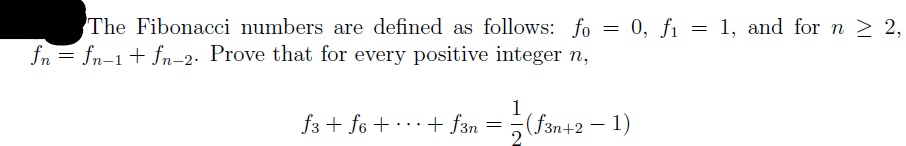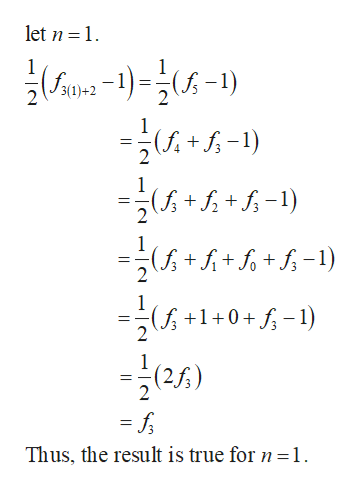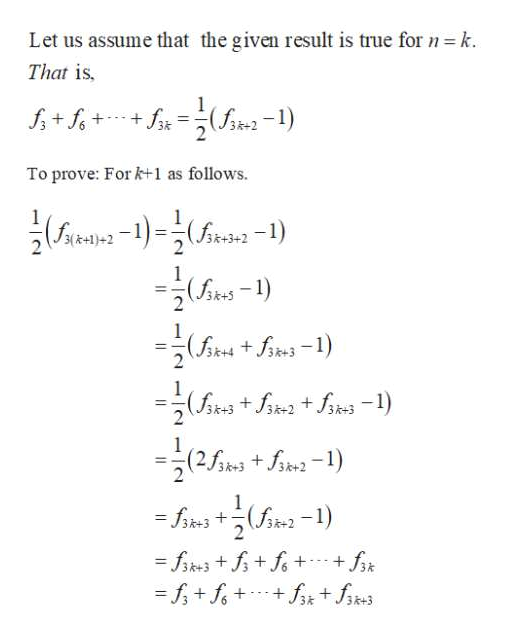# 1. and for n 2 2,The Fibonacci numbers are defined as follows: fo = 0, fı = 1, and for n > 2,fn = fn-1+ fn-2. Prove that for every positive integer n,f3 + fo + ..+ f3n =(f3n+2 – 1)– 1)

Question
4 views

Use Mathematical Induction to provehelp_outlineImage Transcriptionclose1. and for n 2 2, The Fibonacci numbers are defined as follows: fo = 0, fı = 1, and for n > 2, fn = fn-1+ fn-2. Prove that for every positive integer n, f3 + fo + ..+ f3n =(f3n+2 – 1) – 1) fullscreen
check_circle

star
star
star
star
star
1 Rating
Step 1

Initial case:help_outlineImage Transcriptioncloselet n 1 1 3(1)+2 1 (+-) 1 ( ) 1 2 f + f-1) 2 Thus, the result is true for n =1 fullscreen
Step 2

Induction hypot...help_outlineImage TranscriptioncloseLet us assume that the given result is true for n k That is +f(-) f+f To prove: For k+1 as follows. n1) 3(k+1)+2 1 (ik-1) 2 (+-1) 1 (k++-1) 2 2 fsk+fs2-1) -fik3 +(-1) +fk +fikfiks fk3++f+ -f+f+ fullscreen

### Want to see the full answer?

See Solution

#### Want to see this answer and more?

Solutions are written by subject experts who are available 24/7. Questions are typically answered within 1 hour.*

See Solution
*Response times may vary by subject and question.
Tagged in

### Math